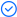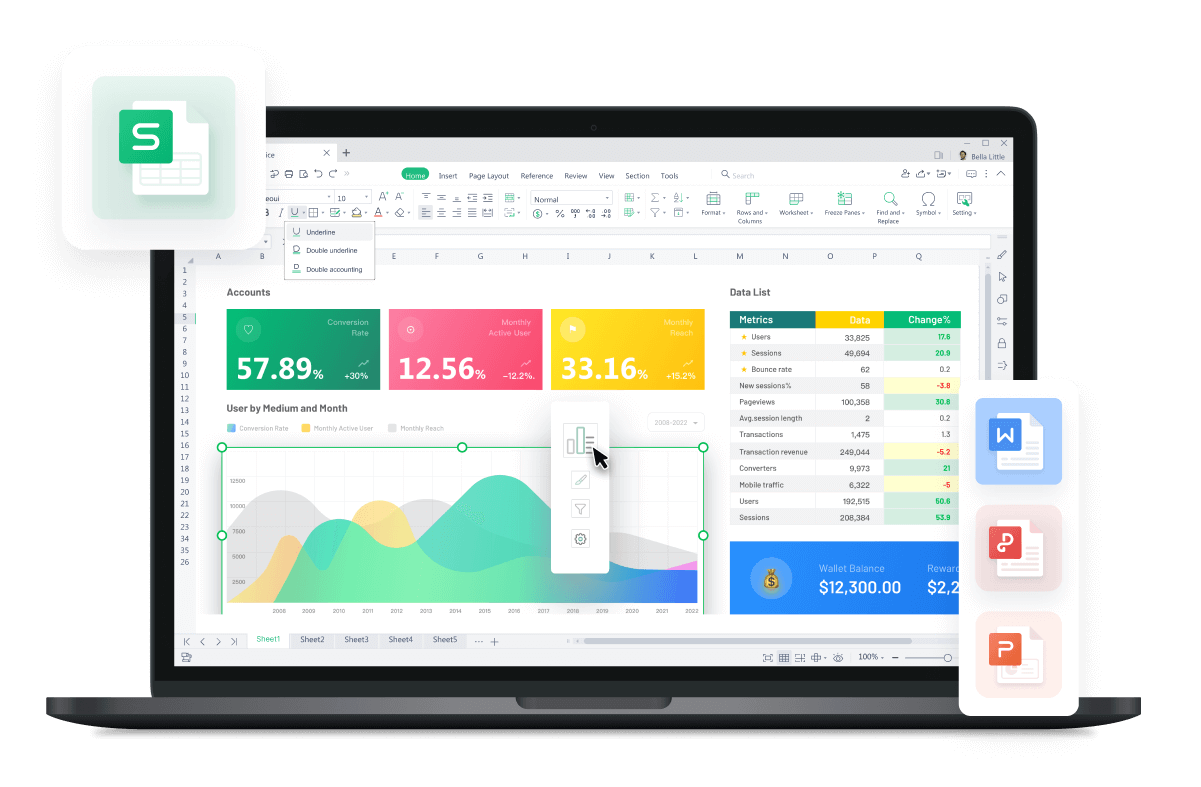WPS Office

Free All-in-One Office Suite with PDF EditorRead, edit, and convert PDFs with the powerful PDF toolkit.Microsoft-like interface, easy to use.

Windows • MacOS • Linux • iOS • Android# How to compare Text in Excel and highlight differences

August 1, 2022
2.9K Views

On occasion, we compare text in  Excel. There are several ways to accomplish this in a single column as well, but there are numerous ways to compare it in Excel to find matches and differences in multiple columns.

Due to Excel's flexibility, there are a number of ways to compare text, including complete comparison and comparison of only a portion of the text using Excel's other functions.

Excel Text Comparison Techniques:

How to compare text in Excel is demonstrated in the technique below.

Approach: 01:Compare text using EXACT Function

It is case sensitive, thus if the two sentences are identical, it will return TRUE. If not, FALSE will be returned.

Using two texts as an example If A1 is the cell and B1 is the cell, then the EXACT function's output will be false.

=EXACT (A1, B1) >> FALSE

Approach: 02: Compare text using Equal Sign (=)

Since it does not worry about case, we should choose this to compare the text when we are not concerned with case. If the two texts don't match, it will return FALSE; if they do, it will return TRUE.

Ex: There are two text strings where A1 has Cell and B1 has cell, then the result of the function will be TRUE.

=EXACT (A1, B1) >> TRUE

Excel Compare Text - First Example

Without regard to the case of the text, two texts—Home city and Preferred City—can be compared in Excel.

Go to Sheet 1 of the Excel sheet that the user wishes to compare in step one.

Step 2: The user wants to compare cities of two different students, so they utilise Excel's formula in the E column to do so.

Step 3: Apply the equal sign (=) by clicking on the E2 column, selecting the B2 cell, and then selecting the D2 cell.

Step 4:  Click the Enter button now.

The outcome of example 1 shows that B2 is in Tokyo and D2 is in Jeddah, which do not match, making the conclusion FALSE.If we look at the fifth row, we can see that the cases of the words in cells B3 and D3 are different since Goa is in B3 and goa is in D3. However, since the equal function is case-insensitive, the result will still be TRUE.

Excel Compare Text: Second example

How does one compare column data in Excel that they wish to match up with text cases?

Step 1: Navigate to Sheet 2 of the Excel workbook where the user wants to compare the data. The user wants to compare the data of cities of two different students, so use the formula in the E column to compare.

Step 2: Click on the E2 cell, then choose the B2 cell, then select D2, all while using the EXACT function.

=EXACT(B2,D2)

Step 3: After pressing the Enter key, the outcome will be shown depending on the data you entered.

An overview of Example #2:

The result of the Excel sheet 2 examples shows that B2 is Tokyo and D2 is Jeddah, which do not match. As a consequence, the result is FALSE.The case of the words in B2 and D2 in the second row's cells differs, but the EXACT function will take this into account because it is case sensitive, hence the result will be FALSE.